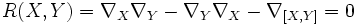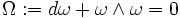# Flat connection

## Definition

### Symbol-free definition

A connection on a vector bundle over a differential manifold is said to be flat or integrable or curvature-free or locally flat if the curvature of the connection is zero everywhere.

### Definition with symbols

A connection$\nabla$ on a differential manifold$M$ is said to be flat or integrable or curvature-free or locally flat if the curvature form vanishes identically, viz for any vector fields$X$ and$Y$:$R(X,Y) = \nabla_X\nabla_Y - \nabla_Y\nabla_X - \nabla_{[X,Y]} = 0$

### Definition in local coordinates

In local coordinates, we require that the curvature matrix should vanish identically; in other words:$\Omega := d\omega + \omega \wedge \omega = 0$

where$\omega$ is the matrix of connection forms.